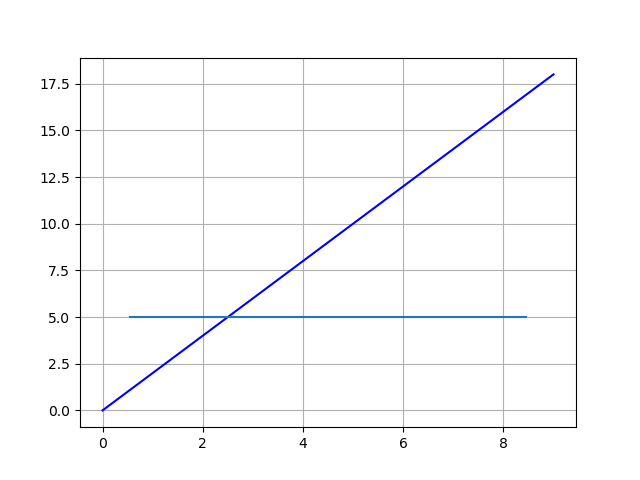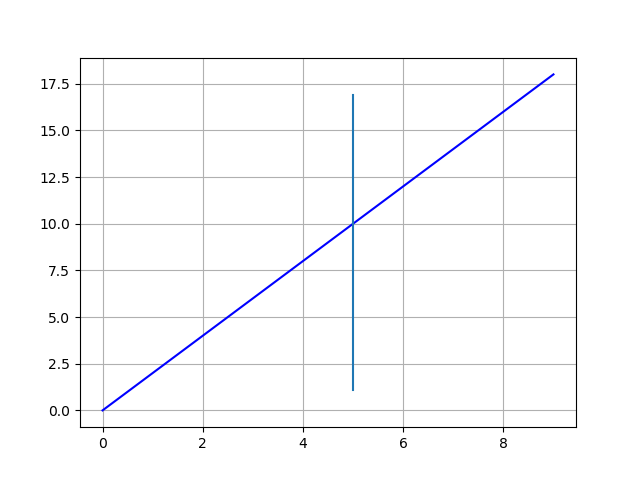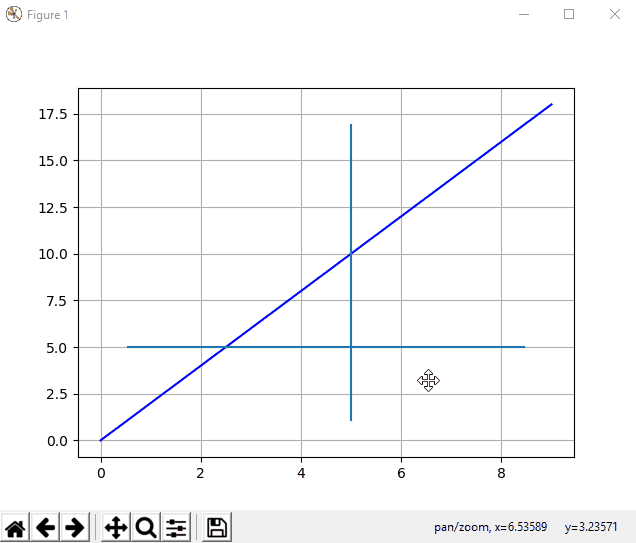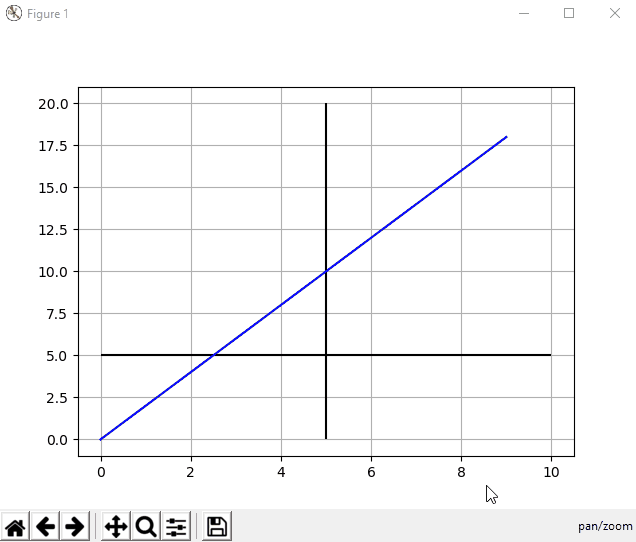# Plot Horizontal and Vertical Line in Matplotlib

Jinku Hu Dec 10, 2020 Nov 24, 2019

In this tutorial, we will introduce two methods to draw horizontal and vertical lines in Matplotlib. These two methods use different coordinates in Matplotlib.

• `axhline()` / `axvline()`
• `hlines()` / `vlines()`

## `axhline` and `axvline` to Plot Horizontal and Vertical Lines in Matplotlib

### `axhline` to Plot a Horizontal Line

``````matplotlib.pyplot.axhline(y=0, xmin=0, xmax=1, hold=None, **kwargs)
``````

`axhline` plots a horizontal line at the position of `y` in data coordinate of the horizontal line, starting from `xmin` to `xmax` that should be between `0.0` and `1.0`, where `0.0` is the far left of the plot and `1.0` is the far right of the plot.

``````from matplotlib import pyplot as plt

xdata = list(range(10))
ydata = [_*2 for _ in xdata]

plt.plot(xdata, ydata, 'b')

plt.axhline(y=5, xmin=0.1, xmax=0.9)

plt.grid()
plt.show()
``````### `axvline` to Plot a Vertical Line

Similarly,

``````matplotlib.pyplot.axvline(x=0, ymin=0, ymax=1, hold=None, **kwargs)
``````

`axvline` plots a vertical line at the position of `x` in data coordinate of the vertical line, starting from `ymin` to `ymax` that should be between `0.0` and `1.0`, where `0.0` is the bottom of the plot and `1.0` is the top of the plot.

``````from matplotlib import pyplot as plt

xdata = list(range(10))
ydata = [_*2 for _ in xdata]

plt.plot(xdata, ydata, 'b')

plt.axvline(x=5, ymin=0.1, ymax=0.9)

plt.grid()
plt.show()
``````As stated above, `xmin` / `xmax` and `ymin` / `ymax` are referred to the plot, but not to the data lines.

Therefore, if we zoom in or out the plot, the starting and ending points of the horizontal and vertical lines will be updated with the reference to the data coordinate, but stick to the relative positions in the plot coordinate. We could check the below animation to have better a understanding.## `hlines` and `vlines` to Plot Horizontal and Vertical Line in Matplotlib

If we want the plotted horizontal and vertical lines will change automatically to keep the relative position to the data coordinate, we need to use `hlines` and `vlines` to plot lines.

``````hlines(y, xmin, xmax)
``````

Here, `y`, `xmin` and `xmax` are the values of the data coordinate.

``````vlines(x, ymin, ymax)
``````

Here, `x`, `ymin` and `ymax` are the values of the data coordinate.

Let’s see the example codes below.

``````from matplotlib import pyplot as plt

xdata = list(range(10))
ydata = [_*2 for _ in xdata]

plt.plot(xdata, ydata, 'b')

plt.hlines(y=5, xmin=0, xmax=10)
plt.vlines(x=5, ymin=0, ymax=20)

plt.grid()
plt.show()
``````## Conclusion of Drawing Horizontal and Vertical Lines in Matplotlib

If you need the line to be referred to the plot, `axhline` and `axvline` should be the better option.

If you prefer the line to stick to the data coordinate, `hlines` and `vlines` are the better choices.

Author: Jinku Hu

Founder of DelftStack.com. Jinku has worked in the robotics and automotive industries for over 8 years. He sharpened his coding skills when he needed to do the automatic testing, data collection from remote servers and report creation from the endurance test. He is from an electrical/electronics engineering background but has expanded his interest to embedded electronics, embedded programming and front-/back-end programming.